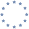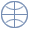Financial mathematicsFinancial Mathematics (also mathematical finance) is the branch of mathematics focused on financial markets. The content of mathematical finance is the development of mathematical models arising from financial economics and their mathematical treatment, e.g. the portfolio theory of Markowitz or the famous Black Scholes option prize formula.

Vocabulary

The list gives an overview of the most important glossaries covering terms which are relevant in financial mathematics.
What is financial mathematics? Find definitions and keywords in:

Subcategories of Mathematical Economics (MSC)

Financial mathematics is a part of mathematical economics 91BXX.

Financial Mathematics in ZBMATH

The database ZBMATH contains references to many articles and books concerning financial mathematics. The classification scheme MSC 2010 can be used to facilitate retrieval of relevant data. Material concerning financial mathematics is classified generally as 91BXX. Selected fields of financial mathematics are:

• Price theory and market structure → ZBMATH
• Finance, portfolios, investments → ZBMATH
• Risk theory and market structure → ZBMATH

Journals and Serials covering Aspects of Financial Mathematics

Below is a link to a list of journals covered by ZBMATH with the main focus on mathematical economics. Please note that in general non-specialized journals for mathematics may also contain articles in financial mathematics.
Journals and Serials in mathematical economics